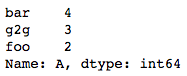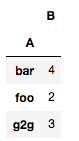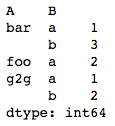# Getting frequency counts of a columns in Pandas DataFrame

Given a Pandas dataframe, we need to find the frequency counts of each item in one or more columns of this dataframe. This can be achieved in multiple ways:

Method #1: Using `Series.value_counts()`

This method is applicable to pandas.Series object. Since each DataFrame object is a collection of Series object, we can apply this method to get the frequency counts of values in one column.

 `# importing pandas as pd ` `import` `pandas as pd ` ` `  `# sample dataframe ` `df ``=` `pd.DataFrame({``'A'``: [``'foo'``, ``'bar'``, ``'g2g'``, ``'g2g'``, ``'g2g'``, ` `                         ``'bar'``, ``'bar'``, ``'foo'``, ``'bar'``], ` `                  ``'B'``: [``'a'``, ``'b'``, ``'a'``, ``'b'``, ``'b'``, ``'b'``, ``'a'``, ``'a'``, ``'b'``] }) ` ` `  `# frequency count of column A ` `count ``=` `df[``'A'``].value_counts() ` `print``(count) `

Output:Method #2: Using `GroupBy.count()`

This method can be used to count frequencies of objects over single columns. After grouping a DataFrame object on one column, we can apply `count()` method on the resulting groupby object to get a DataFrame object containing frequency count.

 `# importing pandas as pd ` `import` `pandas as pd ` ` `  `# sample dataframe ` `df ``=` `pd.DataFrame({ ``'A'``: [``'foo'``, ``'bar'``, ``'g2g'``, ``'g2g'``, ``'g2g'``, ` `                                ``'bar'``, ``'bar'``, ``'foo'``, ``'bar'``], ` `                   ``'B'``: [``'a'``, ``'b'``, ``'a'``, ``'b'``, ``'b'``, ``'b'``, ``'a'``, ``'a'``, ``'b'``] }) ` ` `  `# Multi-column frequency count ` `count ``=` `df.groupby([``'A'``]).count() ` `print``(count) `

Output:Method #3: Using `GroupBy.size()`

This method can be used to count frequencies of objects over single or multiple columns. After grouping a DataFrame object on one or more columns, we can apply `size()` method on the resulting groupby object to get a Series object containing frequency count.

 `# importing pandas as pd ` `import` `pandas as pd ` ` `  `# sample dataframe ` `df ``=` `pd.DataFrame({ ``'A'``: [``'foo'``, ``'bar'``, ``'g2g'``, ``'g2g'``, ``'g2g'``,  ` `                                ``'bar'``, ``'bar'``, ``'foo'``, ``'bar'``], ` `                   ``'B'``: [``'a'``, ``'b'``, ``'a'``, ``'b'``, ``'b'``, ``'b'``, ``'a'``, ``'a'``, ``'b'``] }) ` ` `  `# Multi-column frequency count ` `count ``=` `df.groupby([``'A'``, ``'B'``]).size() ` `print``(count) `

Output:Whether you're preparing for your first job interview or aiming to upskill in this ever-evolving tech landscape, GeeksforGeeks Courses are your key to success. We provide top-quality content at affordable prices, all geared towards accelerating your growth in a time-bound manner. Join the millions we've already empowered, and we're here to do the same for you. Don't miss out - check it out now!

Previous
Next